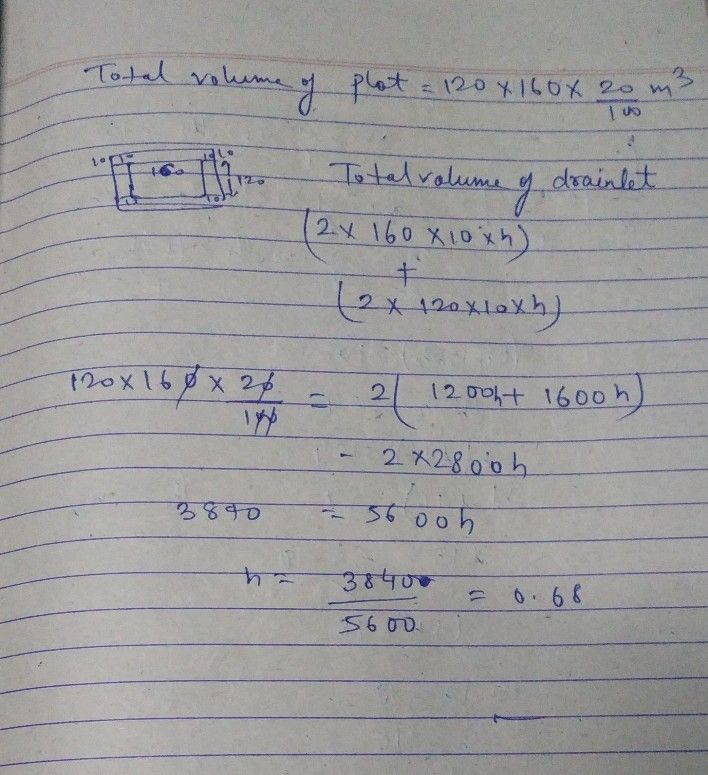Symbol
ProblemWhat equation would you use to solve for $\times 3$ $128^{°}$ $12x^{°}$ $○$ $2x=128$ $O$ $2x+128=100$ $○$ $2x+128=180$ $○$ $2x+2\times =128$